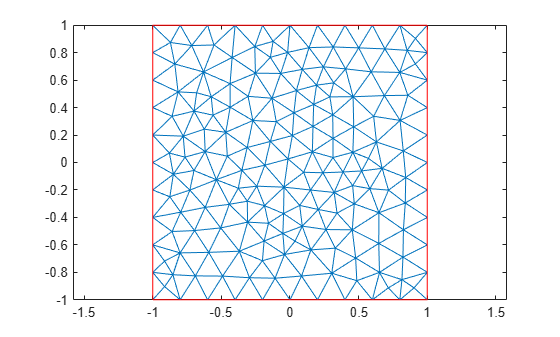# pdetrg

(Not recommended) Triangle geometry data

`pdetrg` is not recommended. Use `area` instead.

## Syntax

``[ar,a1,a2,a3] = pdetrg(p,t)``
``[ar,g1x,g1y,g2x,g2y,g3x,g3y] = pdetrg(p,t)``

## Description

example

````[ar,a1,a2,a3] = pdetrg(p,t)` returns the areas of individual mesh triangles as a vector `ar` and half of the negative cotangent of each angle as vectors `a1,a2,a3`.```

example

````[ar,g1x,g1y,g2x,g2y,g3x,g3y] = pdetrg(p,t)` returns the areas of individual mesh triangles as a vector `ar` and the gradient components of the triangle base functions as vectors `g1x,g1y,g2x,g2y,g3x,g3y`.```

## Examples

collapse all

Find the area of each element of a 2-D mesh. In addition, return half of the negative cotangent of each angle and the gradient components of the triangle base functions for each element.

Generate and plot a mesh for a unit square geometry.

```[p,e,t] = initmesh(@squareg); pdemesh(p,e,t)```Compute the area of each individual element of the mesh and half of the negative cotangent of each angle. Display the first 5 elements for each result.

```[ar,a1,a2,a3] = pdetrg(p,t); ar(1:5)```
```ans = 1×5 0.0126 0.0148 0.0144 0.0156 0.0118 ```
`a1(1:5)`
```ans = 1×5 -0.2819 -0.3905 -0.5332 -0.1812 -0.5237 ```
`a2(1:5)`
```ans = 1×5 -0.5124 -0.2842 -0.1613 -0.4616 -0.3267 ```
`a3(1:5)`
```ans = 1×5 -0.1329 -0.2061 -0.2362 -0.2588 -0.0928 ```

Find the area of the smallest and the largest element of the mesh.

`min(ar)`
```ans = 0.0061 ```
`max(ar)`
```ans = 0.0216 ```

Use the syntax with seven output arguments to compute the gradient components of the triangle base functions for each element.

`[ar,g1x,g1y,g2x,g2y,g3x,g3y] = pdetrg(p,t);`

## Input Arguments

collapse all

Mesh nodes, specified as a 2-by-`Np` matrix of nodes (points), where `Np` is the number of nodes in the mesh. For details on the mesh data representation, see `initmesh`.

Data Types: `double`

Mesh elements, specified as a `4`-by-`Nt` matrix of triangles, where `Nt` is the number of triangles in the mesh. For details on the mesh data representation, see `initmesh`.

Data Types: `double`

## Output Arguments

collapse all

Areas of individual elements, returned as a row vector of positive numbers.

Half of the negative cotangent of each angle, returned as three row vectors of numbers.

Gradient components of the triangle base functions, returned as six row vectors of numbers.

## Version History

Introduced before R2006a

expand all